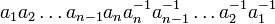# Tour:Inverse map is involutive

This article adapts material from the main article: inverse map is involutive

This page is part of the Groupprops Guided tour for beginners (Jump to beginning of tour)
PREVIOUS: Associative binary operation |UP: Introduction two (beginners) | NEXT: Order of a group
WHAT YOU NEED TO DO:
• Understand the statements below
• Try proving them, using what you understand about associativity and inverses
• Check the proof given in the text
PONDER: What weakening of associativity would be sufficient for the reversal law to continue to hold? (See where precisely associativity is used in the proof)

## Statement

The inverse map in a group, i.e. the map sending any element of the group, to its inverse element, is an involutive map, in the sense that it has the following two properties:

• It satisfies the reversal law:$(a_1a_2 \ldots a_n)^{-1} = a_n^{-1}a_{n-1}^{-1}\ldots a_1^{-1}$

• Applying it twice sends an element to itself:$(a^{-1})^{-1} = a$

It fact, both these are true in the greater generality of a monoid, under the condition that all the$a_i$s have two-sided inverse$a_i^{-1}$ (note: we still need a monoid to guarantee that two-sided inverses, when they exist, are unique).

## Proof

### Proof of reversal law

In order to show that the element$a_n^{-1}a_{n-1}^{-1}\ldots a_1^{-1}$ is a two-sided inverse of$a_1a_2 \ldots a_n$, it suffices to show that their product both ways is the identity element. Consider first the product:$(a_1a_2 \ldots a_{n-1}a_n)(a_n^{-1}a_{n-1}^{-1}\dots a_2^{-1}a_1^{-1})$

Due to associativity, we can drop the parentheses and we get:$a_1a_2 \ldots a_{n-1}a_na_n^{-1}a_{n-1}^{-1}\dots a_2^{-1}a_1^{-1}$

Now, consider the middle product$a_na_n^{-1}$. This is the identity element, and since the identity element has no effect on the remaining product, it can be removed, giving the product:$a_1a_2 \ldots a_{n-1}a_{n-1}^{-1}\dots a_2^{-1}a_1^{-1}$

We now repeat the argument with the middle product$a_{n-1}a_{n-1}^{-1}$ and cancel them. Proceeding this way, we are able to cancel all terms and eventually get the identity element.

A similar argument follows for the product the other way around:$(a_n^{-1}a_{n-1}^{-1}\ldots a_1^{-1})(a_1a_2 \ldots a_n)$

Thus, the elements are two-sided inverses of each other.

Note: In fact, it suffices to check only one of the two inverse conditions, i.e., check only that the first product is the identity element. This is because, in a group, every element has a two-sided inverse. Further, equality of left and right inverses in monoid forces any one-sided (left or right) inverse to be equal to the two-sided inverse.

### Proof for applying it twice

This is direct from the definition. let$b = a^{-1}$. Then, by the inherent symmetry in the definition of inverse element, we see that$a = b^{-1}$.

More explicitly, if$b = a^{-1}$, that means that$ab = ba = e$. But this is precisely the condition for stating that$a = b^{-1}$.

This page is part of the Groupprops Guided tour for beginners (Jump to beginning of tour). If you found anything difficult or unclear, make a note of it; it is likely to be resolved by the end of the tour.
PREVIOUS: Associative binary operation | UP: Introduction two (beginners) | NEXT: Order of a group